# 口算题卡三年级上册RJ答案下载-五E口算题卡三年级上册电子版免费版

### 五E口算题卡三年级上册图片预览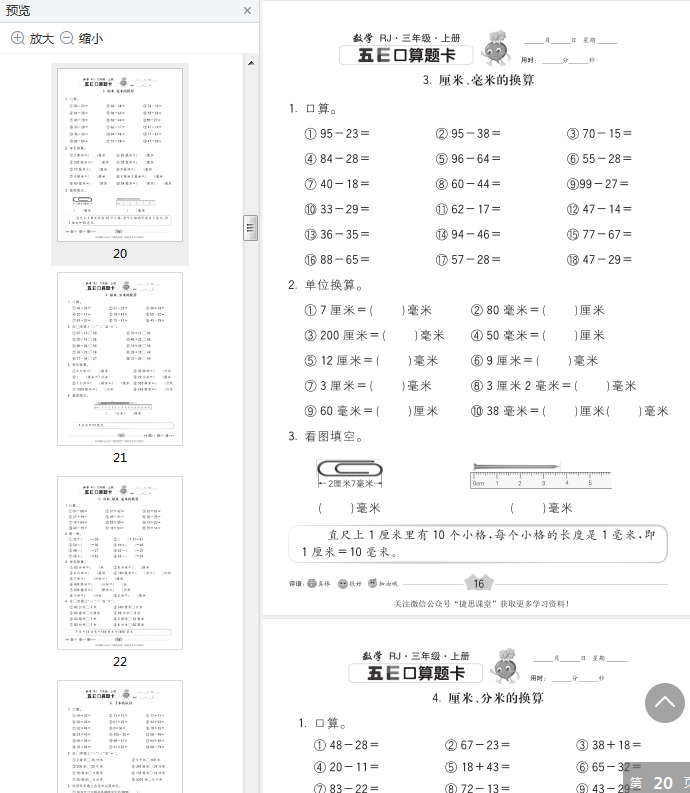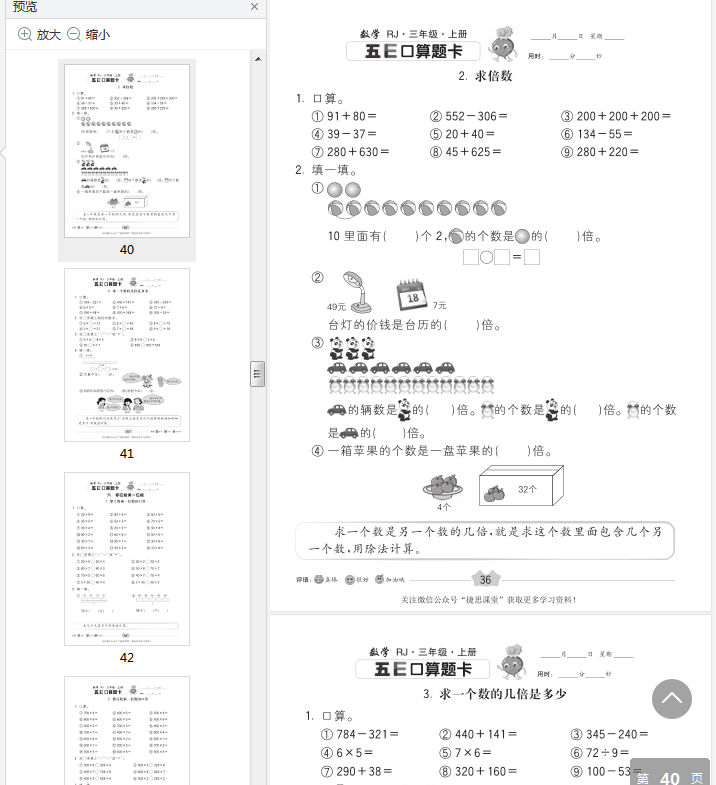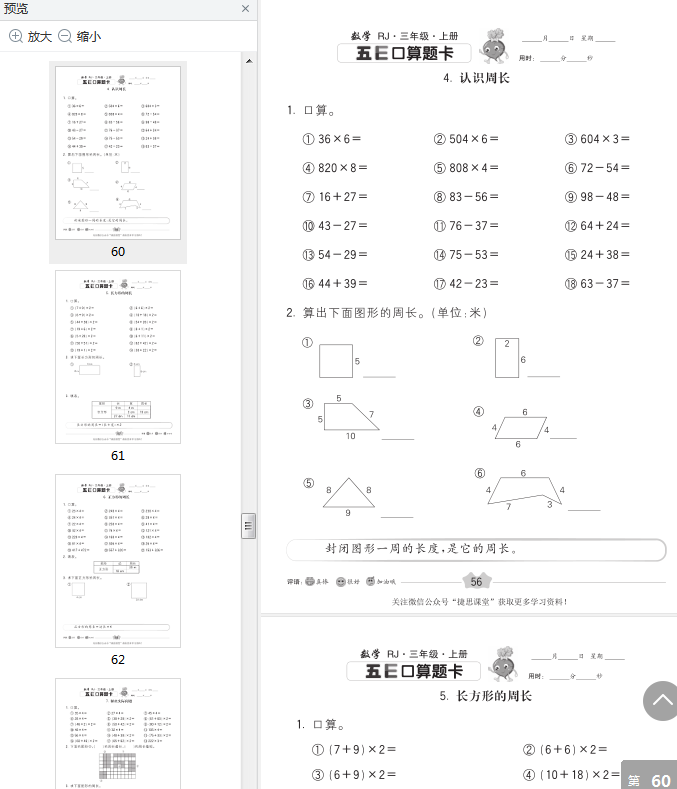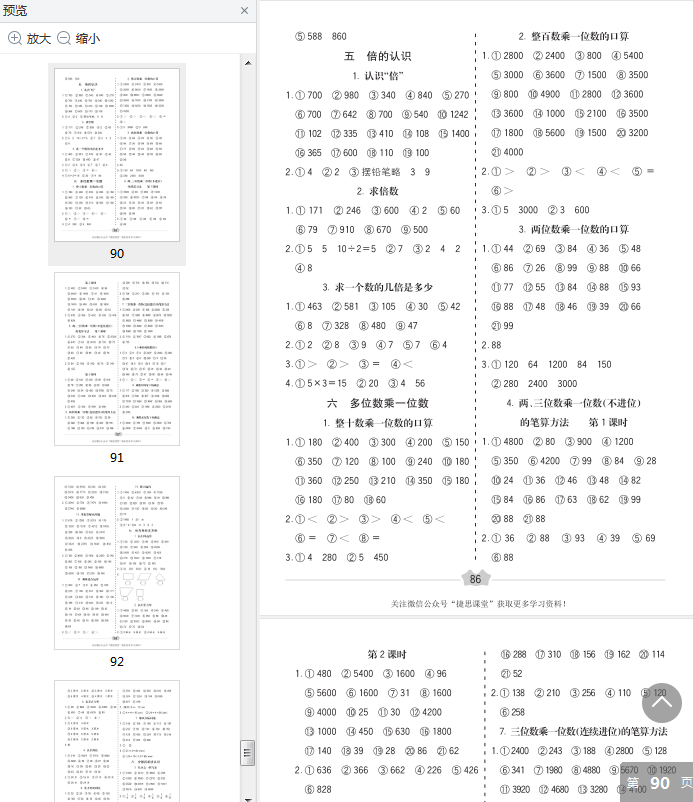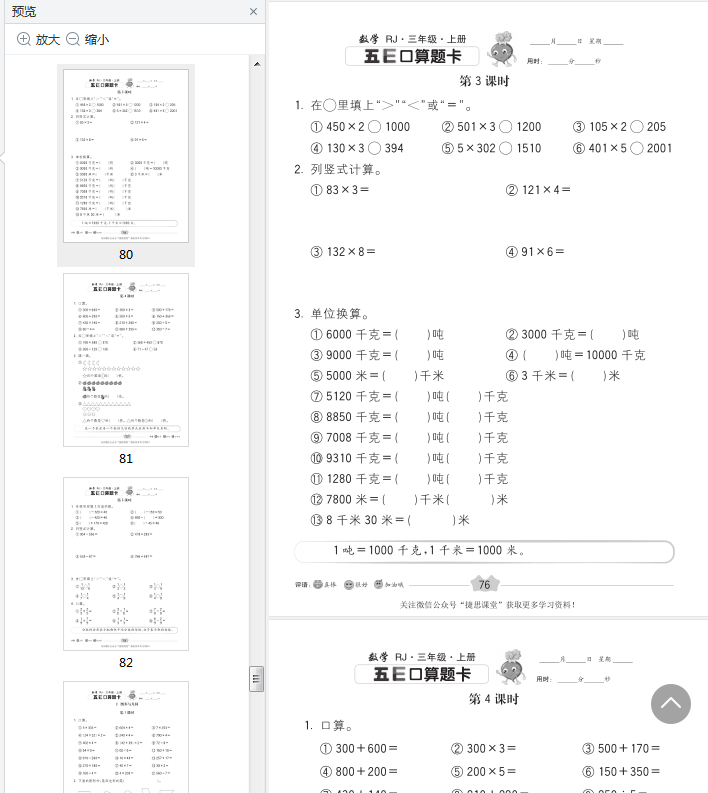### 五E口算题卡三年级上册目录部分

1，秒和分的关系.2.时和分的换算…

3，时、分、秒的换算第1课时.

4.经过的时间

2，两位数加两位数（进位）..

3，两位数减两位数（不退位）

4，两位数减两位数（退位）….

5.几百几十加几百Л+6．几百几十减几百几十7，三位数的近似数8，三位数加减法的估算..

1，毫米的认识2.分米的认识

3，厘米、毫米的换算…

4，厘米、分米的换算

5.分米、厘米、毫米的换算

6.千米的认识

7.千米、米的换算.

8．距离的估算9.吨的认识

10，解决问题的策略（列表法）

1，三位数加三位数（不进位）

2.三位数加三位数（一次进位）3.三位数加三位数（连续进位）

4.三位数加三位数的验算15.三位数减法（不退位）

6.三位数减法（连续退位）…

7.三位数减三位数（被减数中间有.这…..

8、整百数减三位数的连续退位减法9·三位数减法的验算10，解决问题..

1，认识“倍"…

2.求倍数..

3.求一个数的几倍是多少六多位数乘一位数

1，整十数乘一位数的口算2.整百数乘一位数的口算3.两位数乘一位数的口算4、两、三位数乘一位数（不进位）的笔算方法第1课时第2课时

### 口算题卡三年级上册RJ答案130-27=

7×800=

56-8=

146-23=

24:3=

500×2=

700×4=

546-457=

40×7=

72:8=

1800-800=

33+57=

63-7=

800+8=

36:4=

56+7=

879+54=

7×700=

31×3=

457+456=

2×330=

84+50=

660-90=

7+14=

4300-700=

6×800=

63:3=

600×3=

62-33=

0:800=

24:3=

86-49=

95-20=

84-9=

13×3=

100-21=

50×6=

62+48=

25+55=

105-22=

86-24=

60-9=

26+57=

37+8=

500+500=

0:47=

24×2=

300×8=

60-13=

430×2=

34+56=

65-14=

68:4=

72+19=

34+56=

70-15=

45+37=

1500-900=

80-8=

45-5=

33×2=

56-7=

0:54=

33-25=

### 五E口算题卡三年级上册电子版免费版截图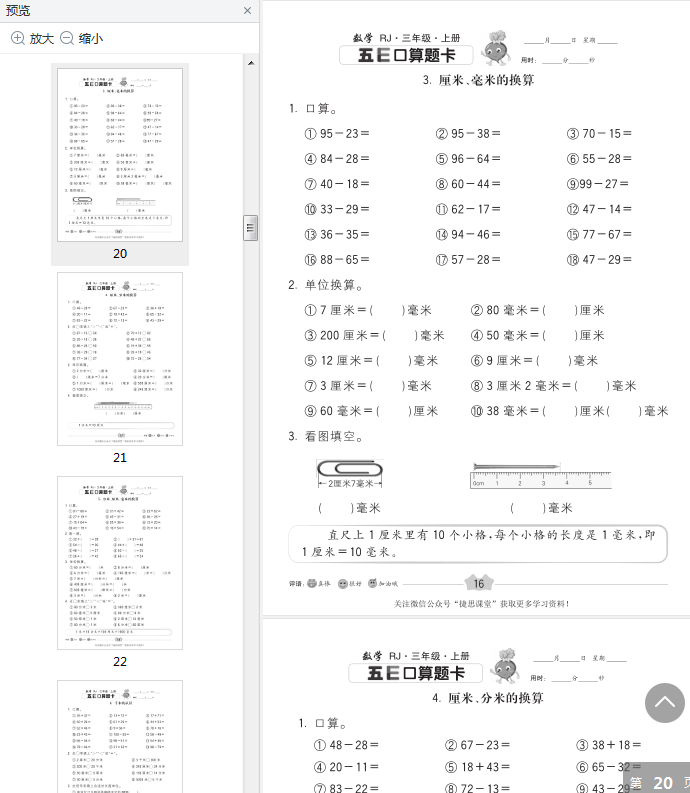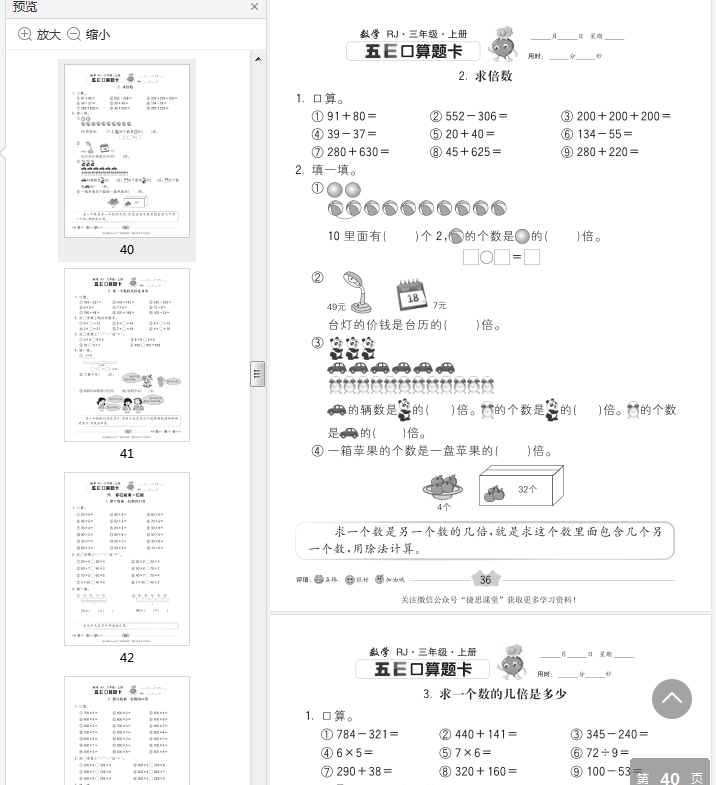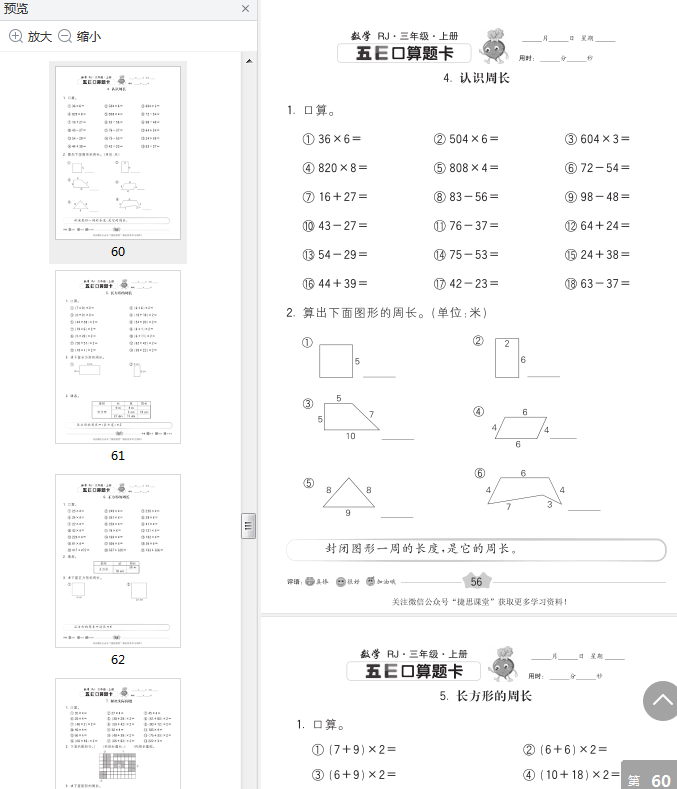• 5 分
0
• 4 分
0
• 3 分
0
• 2 分
0
• 1 分
0